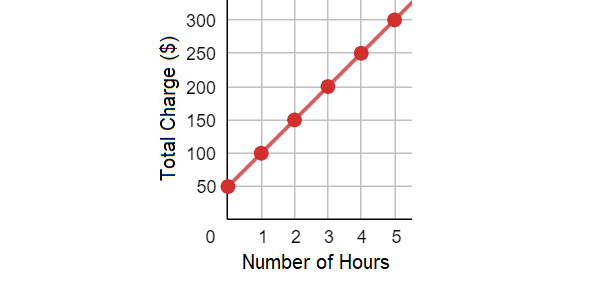Question# The equation a=50h+50 represents the amount a that an air-conditioning repair company charges for h hours of labour. Make a table and sketch a graph of the equation

Analyzing categorical data
ANSWEREDThe equation $$a=50h+50$$ represents the amount a that an air-conditioning repair company charges for h hours of labour. Make a table and sketch a graph of the equation2021-02-26

To make a table, substitute different values of hh into $$a=50h+50$$.

Since hh represents time, which can't be negative, only substitute values of hh such that

$$h\geq0h\geq0: h | 50h+50 | (h,a) 0 | 50(0)+50$$

$$=50 | (0,50) 1 | 50(1)+50$$

$$=100 | (1,100) 2 | 50(2)+50$$

$$=150 | (2,150) 3 | 50(3)+50$$

$$=200 | (3,200) 4 | 50(4)+50$$

$$=250 | (4,250) 5 | 50(5)+50$$

$$=300 | (5,300)$$

Plot the points from your table and then draw a line passing through the points to sketch the graph of the equation. Then label the vertical axis as Total Charge (\$) and the horizontal axis as Number of Hours: